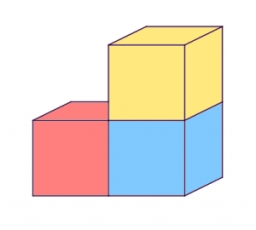# One-fifth 17753

The store stacked two hundred boxes of washing powder in three piles. There were 13 more boxes in the first pile than in the second pile, one-fifth more in the second pile than in the third pile. How many boxes were in which pile?

a =  79
b =  66
c =  55

### Step-by-step explanation:

200=a+b+c
a = 13+b
b = c+c/5

a+b+c = 200
a-b = 13
5b-6c = 0

Row 2 - Row 1 → Row 2
a+b+c = 200
-2b-c = -187
5b-6c = 0

Pivot: Row 2 ↔ Row 3
a+b+c = 200
5b-6c = 0
-2b-c = -187

Row 3 - -2/5 · Row 2 → Row 3
a+b+c = 200
5b-6c = 0
-3.4c = -187

c = -187/-3.4 = 55
b = 0+6c/5 = 0+6 · 55/5 = 66
a = 200-b-c = 200-66-55 = 79

a = 79
b = 66
c = 55

Our linear equations calculator calculates it.Did you find an error or inaccuracy? Feel free to write us. Thank you!

Tips for related online calculators
Do you have a linear equation or system of equations and looking for its solution? Or do you have a quadratic equation?